# Almost Periodic Function

(redirected from Almost-periodic)
The following article is from The Great Soviet Encyclopedia (1979). It might be outdated or ideologically biased.

## Almost Periodic Function

a function whose value is approximately repeated when its argument is increased by properly selected constants (the almost periods). More precisely, a continuous function f(x) defined for all real values of 5 x is called almost periodic if for every > 0 there exists an l = l (∊) such that in every interval of length l on the x-axis at least one number τ = τ(∊) can be found for which the inequality ǀf (x + τ) – f(x)ǀ < ∊ is satisfied for all x. The numbers τ are called the almost periods of the function f(x). Periodic functions are special cases of almost periodic functions; simple examples of almost periodic functions that are not periodic can be obtained by adding periodic functions with incommensurable periods—for example, cos x + cos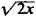.

The following are some important properties of almost periodic functions:

(1) An almost periodic function is bounded and uniformly continuous on the entire x-axis.

(2) The sum and product of a finite number of almost periodic functions is an almost periodic function.

(3) The limit of a uniformly convergent sequence of almost periodic functions is an almost periodic function.

(4) Every almost periodic function has a mean value (over the entire x-axis):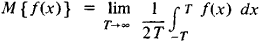(5) To every almost periodic function we can associate a Fourier series: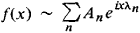where λ1, λ2, …, λn, … can be any sequence of distinct real numbers and

An = M {f (x)enx}

(6) The Parseval equality: for every almost periodic function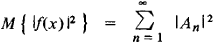(7) Uniqueness theorem: if f(x) is a continuous almost periodic function and if for all real λ

M {f (x)eiλx} = 0

then f(x) ≡ 0. In other words, a Fourier series uniquely determines an almost periodic function.

(8) Approximation theorem: for every ∊ > 0, there exists a finite trigonometric polynomial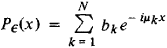(where μκ is a real number) such that the inequality ǀf(x) – P (x)ǀ < ∊ is satisfied for all values of x; conversely, every function f(x) with this property is an almost periodic function.

The first construction of almost periodic functions was given by the Danish mathematician H. Bohr in 1923. Even earlier, in 1893, the Latvian mathematician P. Bohl studied a special case of almost periodic functions—quasi-periodic functions. A new construction of the theory of almost periodic functions was provided by N. N. Bogoliubov in 1930. The theory of almost periodic functions was generalized to include discontinuous functions first by V. V. Stepanov in 1925 and subsequently by H. Weyl and A. S. Besicovitch. A generalization of a different kind was given by the Soviet mathematician B. M. Levitan in 1938.

### REFERENCES

Bohr, H. Pochti periodicheskie funktsii. Moscow-Leningrad, 1934. (Translated from German.)
Levitan, B. M. Pochti-periodicheskie funktsii. Moscow, 1953.
References in periodicals archive ?
Prouse, Almost-Periodic Functions and Functional Equations, Van Nostrand-Reinhold, New York, NY, USA, 1971.
Zaidman, Almost-Periodic Functions in Abstract Spaces, vol.
Prouse, Almost-periodic functions and functional equations, Van Nostrand Reinhold Co., New York Toronto, Ont.-Melbourne, 1971.
This was shown in  basing on the fact (the classical result proved in ) that every point x [member of] X is almost-periodic under [phi], if X is a compact metric space and X is minimal for [phi] (the set is minimal if it is non-empty, closed, invariant and such that no proper subset of it shares all these properties).
Rudowski, "The coexistence of periodic, almost-periodic and chaotic attractors in the van der Pol-Duffing oscillator," Journal of Sound and Vibration, vol.
Li, Composition of pseudo almost-periodic functions and semilinear differential equations.
Gordillo, "Robust Generation of Almost-Periodic Oscillations in a Class of Nonlinear Systems," Int.
MANTICA, Wave propagation in almost-periodic structures, Physica D, 109 (1997), pp.
The 31 papers explore such aspects of difference equations as a condition for transitivity of Lorenz maps, invariant manifolds as pullback attractors on non-autonomous difference equations, solutions bounded on the whole line for perturbed difference systems, rational third-order difference equations, some discrete nonlinear inequalities and applications to boundary value problems, the bifurcation of almost-periodic solutions in a difference equation, and oscillation theorems for a class of fourth-order nonlinear difference equations.
It is easy to check that, for X in CCR ([C.sup.n]), X\(*) is almost-periodic (uniformly approximable by linear combinations of characters).
Chen, "Multistability and new attraction basins of almost-periodic solutions of delayed neural networks," IEEE Transactions on Neural Networks, vol.

Site: Follow: Share:
Open / Close